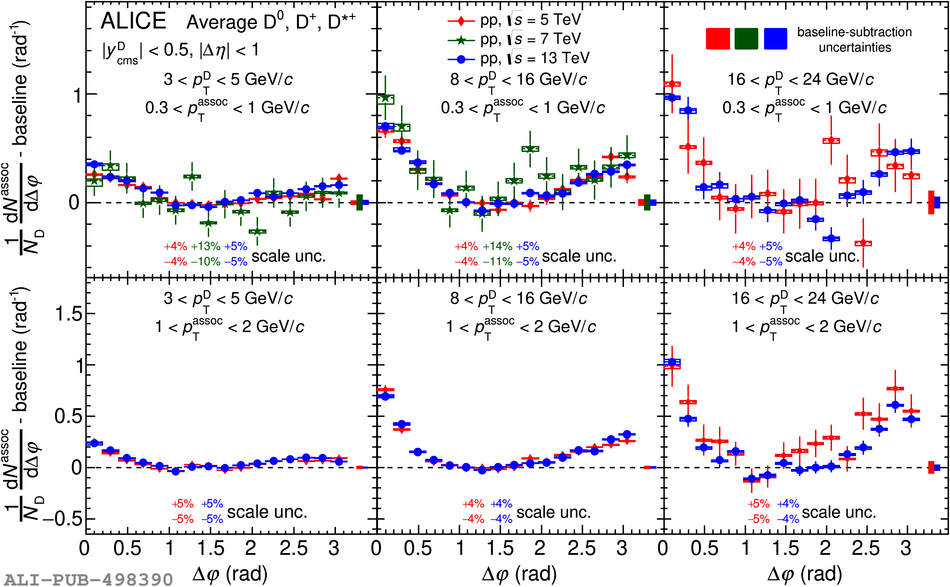Figure 1

 Average of the azimuthal-correlation functions of $\Dzero$, $\Dplus$, and $\Dstar$ mesons with associated particles, after the baseline subtraction, in pp collisions at $\sqrt{s}$ = 5.02~, 7~, and 13~TeV, for 3 $\pi$.